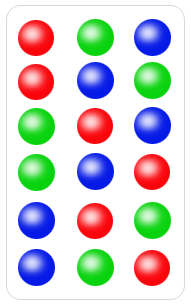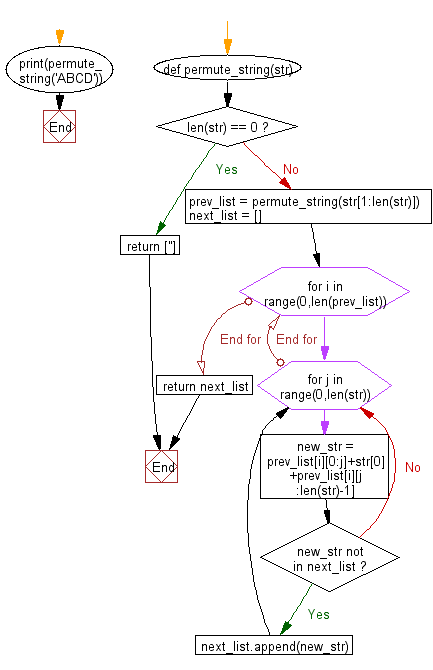﻿ Python Math: Print all permutations of a given string - w3resource# Python Math: Print all permutations of a given string

## Python Math: Exercise-16 with Solution

Write a Python program to print all permutations of a given string (including duplicates).

In mathematics, the notion of permutation relates to the act of arranging all the members of a set into some sequence or order, or if the set is already ordered, rearranging (reordering) its elements, a process called permuting. These differ from combinations, which are selections of some members of a set where order is disregarded.

In the following image each of the six rows is a different permutation of three distinct ballsSample Solution:-

Python Code:

``````def permute_string(str):
if len(str) == 0:
return ['']
prev_list = permute_string(str[1:len(str)])
next_list = []
for i in range(0,len(prev_list)):
for j in range(0,len(str)):
new_str = prev_list[i][0:j]+str+prev_list[i][j:len(str)-1]
if new_str not in next_list:
next_list.append(new_str)
return next_list

print(permute_string('ABCD'));
```
```

Sample Output:

```['ABCD', 'BACD', 'BCAD', 'BCDA', 'ACBD', 'CABD', 'CBAD', 'CBDA', 'ACDB', 'CADB', 'CDAB', 'CDBA', 'ABDC', 'BADC
```

Flowchart:## Visualize Python code execution:

The following tool visualize what the computer is doing step-by-step as it executes the said program:

Python Code Editor:

Have another way to solve this solution? Contribute your code (and comments) through Disqus.

What is the difficulty level of this exercise?

Test your Programming skills with w3resource's quiz.

﻿

```>>> students = [{'name': 'John', 'score': 98}, {'name': 'Mike', 'score': 94}, {'name': 'Jennifer', 'score': 99}]